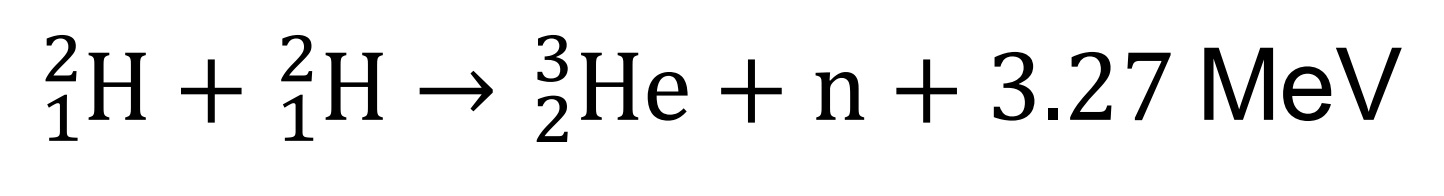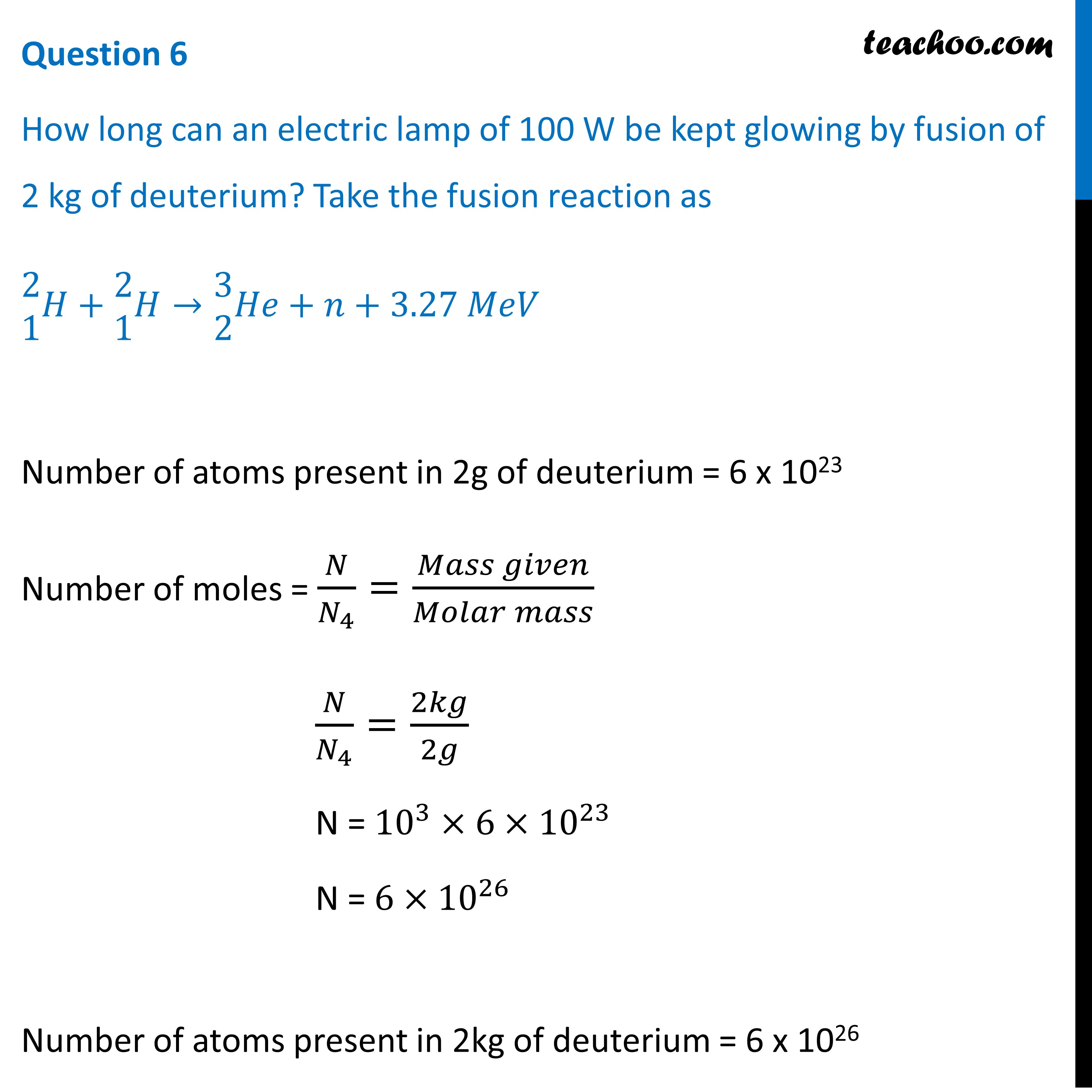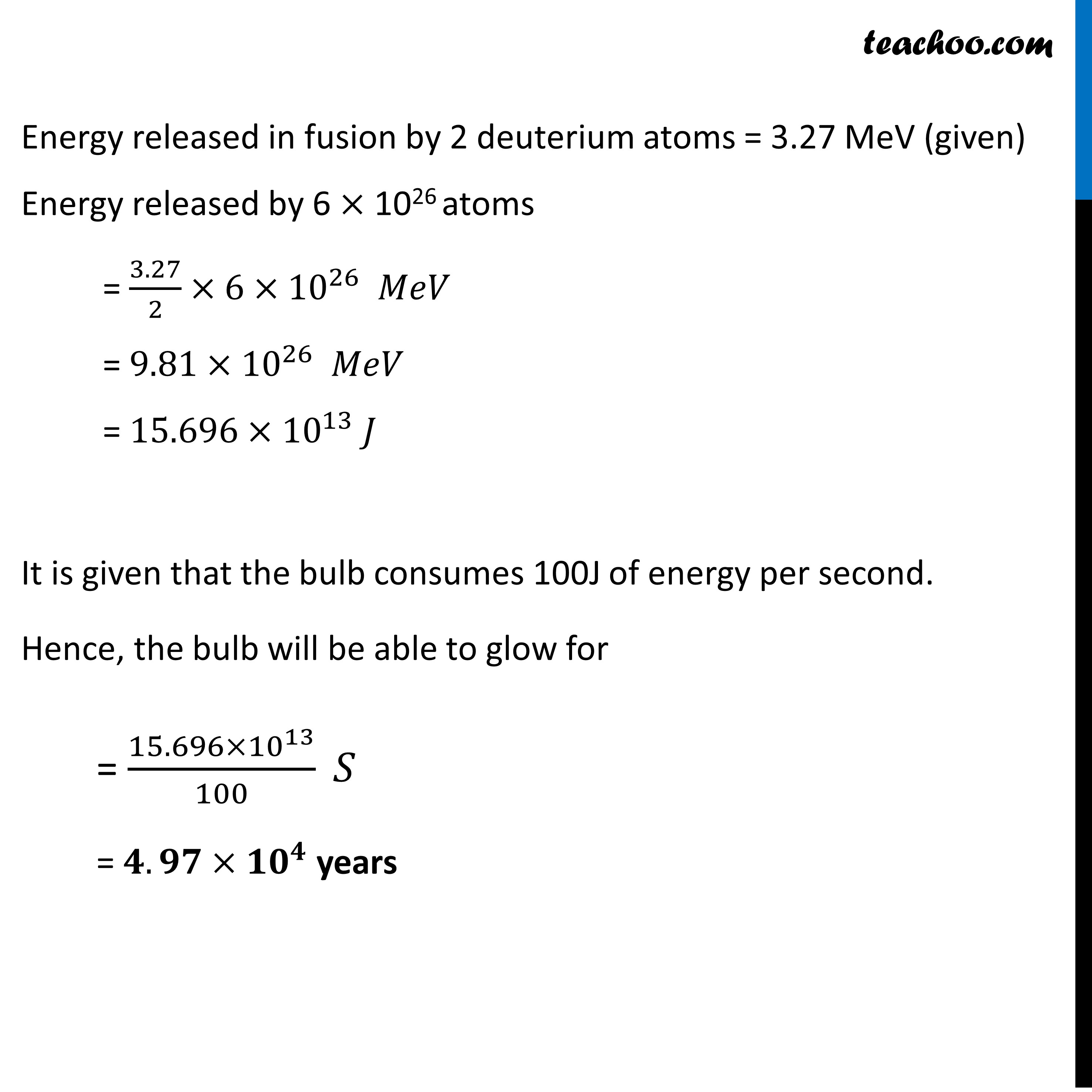CBSE Class 12 Sample Paper for 2022 Boards [Term 2] - Physics

Physics Class 12
Solutions to CBSE Sample Paper - Physics Class 12

## How long can an electric lamp of 100 W be kept glowing by fusion of 2 kg of deuterium? Take the fusion reaction asLearn in your speed, with individual attention - Teachoo Maths 1-on-1 Class

### Transcript

How long can an electric lamp of 100 W be kept glowing by fusion of 2 kg of deuterium? Take the fusion reaction as ■8([email protected])𝐻+■8([email protected])𝐻→■8([email protected])𝐻𝑒+𝑛+3.27 𝑀𝑒𝑉 Question 6 How long can an electric lamp of 100 W be kept glowing by fusion of 2 kg of deuterium? Take the fusion reaction as ■8([email protected])𝐻+■8([email protected])𝐻→■8([email protected])𝐻𝑒+𝑛+3.27 𝑀𝑒𝑉 Number of atoms present in 2g of deuterium = 6 x 1023 Number of moles = 𝑁/𝑁_4 =(𝑀𝑎𝑠𝑠 𝑔𝑖𝑣𝑒𝑛)/(𝑀𝑜𝑙𝑎𝑟 𝑚𝑎𝑠𝑠) 𝑁/𝑁_4 =2𝑘𝑔/2𝑔 N = 10^3×6×10^23 N = 6×10^26 Number of atoms present in 2kg of deuterium = 6 x 1026 Energy released in fusion by 2 deuterium atoms = 3.27 MeV (given) Energy released by 6 × 1026 atoms = 3.27/2×6×10^26 𝑀𝑒𝑉 = 9.81×10^26 𝑀𝑒𝑉 = 15.696×10^13 𝐽 It is given that the bulb consumes 100J of energy per second. Hence, the bulb will be able to glow for = (15.696×10^13)/100 𝑆 = 𝟒.𝟗𝟕×〖𝟏𝟎〗^𝟒 years